## Wednesday, September 30, 2009

### 957

957 = 3 x 11 x 29, which makes 957 a sphenic number. A sphenic number is a positive integer that is the product of three distinct prime numbers.

957 is 12312 in base 5, 4233 in base 6, and 2535 in base 7.

957 is the product of three distinct primes such that the sum of the three primes is also a prime: 3 + 11 + 29 = 43, a prime.

957 is a number such that its base 2 (binary) representation is the juxtaposition of two identical strings: 1110111101.The asteroid 957 Camelia was discovered in 1921.

Source: Positive Integers

## Tuesday, September 29, 2009

### 164

164 = 22 x 41.

164 is the smallest concatenation of two squares in two ways: 1 and 64; 16 and 4.

164 is 20002 in base 3 and 202 in base 9.

164 has a representation as a sum of two squares: 164 = 82 + 102.

164 is a divisor of 812 - 1.164 is the highest possible score of a word in the game Scrabble: playing “Quartzy” across triple-word square with “z” on a double-letter square and a premium of 50 for using all seven letters on the rack.

Source:
Number Gossip

## Monday, September 28, 2009

### 201

201 = 3 x 67.

201n + 2 is a prime number, for n = 14, 15, and 16.

The square of
201 is one of four square numbers using the same digits: 1022 = 10404, 1202 = 14400, 2012 = 40401, and 2102 = 44100.

201 is a Niven (or Harshad) number, which is divisible by the sum of its digits.201 Penelope is a large asteroid discovered in 1879.

Source:
Prime Curios!

## Friday, September 25, 2009

### 302

302 = 2 x 151.

The square of
302 is one of three square numbers using the same digits: 1482 = 21904, 2032 = 41209, and 3022 = 91204.

302 is the number of ways to play the first three moves in checkers.

302 is a number n such that n and n + 1 have the same number of divisors: 303 = 3 x 101.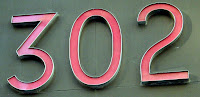302 is the telephone area code for the state of Delaware.

Source:

## Thursday, September 24, 2009

### 503

503 is a prime number.

503 is the smallest prime that is the sum of the cubes of the first n primes: 23 + 33 + 53 +73 = 503.

5! + 0! + 3! is a three-digit Mersenne prime.

503 is a number that cannot be written as a sum of three squares.503 is Clark Kent's Metropolis apartment number in the "Superman" series.

Source: Prime Curios!

## Wednesday, September 23, 2009

### 905

905 = 5 x 181.

Most odd integers can be written as the sum of a prime and a power of two in at least one way. Ones that cannot are called obstinate numbers. They form an infinite set but are not all that common. The first composite obstinate number is 905. So 905 is the smallest composite number that is not the sum of a prime and a power of two.

905 is the sum of seven consecutive primes: 109 + 113 + 127 + 131 + 137 + 139 + 149 = 905.

905 has two representations as a sum of two squares: 905 = 82 + 292 = 112 + 282.

905 is the hypotenuse of two Pythagorean triples: 9052 = 4642 + 7772 = 6162 + 6632.A Neptune-Pluto conjunction occurred in Taurus in the year 905.

Source: Prime Curios!

## Tuesday, September 22, 2009

### 889

889 = 7 x 127. It is a product of distinct Mersenne primes.

889 is an odious number because it has an odd number of ones in its binary expansion: 1101111001.

889 is a number such that the sum of its divisors is a square: 1 + 7 + 127 + 889 = 135 = 32 x 32.

889 is the sum of eight positive fifth powers: 889 = 35 + 35 + 35 + 25 + 25 + 25 + 25 + 25.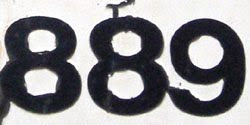In the Dewey Decimal System for classifying books, 889 is the code for literature in medieval and modern Greek

Source: Number Gossip

## Monday, September 21, 2009

### 68

68 = 22 x 17.

68 is the smallest composite number that becomes prime when turned upside down: 89.

68 is the largest even number that is expressible as the sum of two primes in two different ways: 7 + 61 = 68 and 31 + 37 = 68.

68 has a representation as a sum of two squares: 68 = 22 + 82.

68 is 44 in base 16.The chemical element erbium has atomic number 68.

Source: Prime Curios!

## Friday, September 18, 2009

### 1117

1117 is a prime number.

1117 has a representation as a sum of two squares: 1117 = 212 + 262.

1117 is the hypotenuse of a primitive Pythagorean triple: 11172 = 2352 + 10922.

1117 is the smallest four-digit unholey prime: Such primes do not have holes in their digits.

1117 is the smallest four-digit prime comprised of two two-digit primes."One Thousand One Hundred Seventeen" is the smallest number whose English name is spelled with exactly thirty letters.

Source:
Number Gossip

## Thursday, September 17, 2009

### 681

681 = 3 x 227.

The sum of the digits of 681 is five times the number of digits: 6 + 8 + 1 = 15 = 3 x 5.

681 is the sum of 11 positive 5th powers: 681 = 35 + 35 + 25 + 25 + 25 + 25 + 25 + 25 + 15 + 15 + 15.681 is a centered pentagonal number.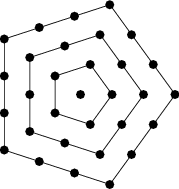The galaxy NGC 681, more than 55 million light years away, resembles the striking Sombrero Galaxy (M104).

Source: Wolfram MathWorld

## Wednesday, September 16, 2009

### 414

414 = 2 x 32 x 23

414 is a value of n for which n4, n5, n6, and n7 have the same digit sum.

4142 = 171,396 = (17 + 1 + 396)2.

414 is 636 in base 8.414 is the telephone area code for Milwaukee, Wisconsin.

## Tuesday, September 15, 2009

### 555

555 = 3 x 5 x 37.

555 is a spheric number. It is the product of three distinct prime numbers.

555 is 2323 in base 6 and 676 in base 9.

The sum of the digits of 555 is five times the number of digits: 5 + 5 + 5 = 3 x 5 = 15.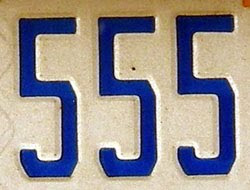In North America, telephone numbers with the prefix 555 are widely used for fictitious telephone numbers in television shows, films, computer games, and other media.

Source: Wikipedia

## Monday, September 14, 2009

### 825

825 = 3 x 52 x 11.

825 is the number of legal ways to add two sets of parentheses to a product of nine variables.

825 is a Smith number. The sum of its digits is equal to the sum of the digits in its prime factorization: 8 + 2 + 5 = 3 + 5 + 5 + 1 + 1.

825 is the sum of six positive fifth powers: 825 = 35 + 35 + 35 + 25 + 25 + 25.Asteroid 825 Tanina was discovered in 1916 and has a diameter of about 11 kilometers.

## Friday, September 11, 2009

### 268

268 = 22 x 67.

268 has a unique representation as a sum of three squares: 268 = 62 + 62 + 142.

The product of
268 and the sum of its digits plus one is prime: 268 * (2 + 6 + 8) + 1 = 4289, a prime.

268 is the smallest number whose product of digits is six times the sum of its digits: 2 * 6 * 8 = 6(2 + 6 + 8).

268 is 414 in base 8.268 inches of 1/8-inch copper wire weigh 1 pound.

Source:
Prime Curios!

## Thursday, September 10, 2009

### 937

937 is a prime number.

937 in base 3 is palindromic: 1021201.

937 has a representation as a sum of two squares: 937 = 192 + 242.

937 is the hypotenuse of a primitive Pythagorean triple: 937 = 2152 + 9122.937 is a star number. A star number is a centered figurate number that represents a centered hexagram, such as the one (below) that Chinese checkers is played on.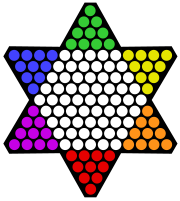Telephone area code 937 covers much of the southwestern part of the state of Ohio.

Source: Wikipedia

## Wednesday, September 9, 2009

### 999

999 = 33 x 37.

999 is the smallest possible sum of three-digit primes that together include all nine digits: 149 + 263 + 587 = 999.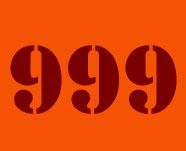The odd powers of 999 end with 999, and its even powers end with 001: 9992 = 998001; 9993 = 997002999.

999 is 4343 in base 6, 2625 in base 7, and 1747 in base 8.

999 is the Dewey Decimal Classification system call number for library books on the general history of extraterrestrial worlds.

Source: Numeropedia

## Tuesday, September 8, 2009

### 789

789 = 3 x 263.

789 is the sum of three consecutive primes: 257 + 263 + 269 = 789.

789 are the first three digits of 9789.

789 occurs in the sequence generated by starting with 1, then repeatedly reversing the digits and adding 2: 1, 3, 5, 7, 9, 11, 13, 33, . . . , 787, 789, 989, . . .In the Year 789, Charlemagne introduced the royal foot as the unit of length.

## Friday, September 4, 2009

### 177

177 = 3 x 59.

177 is a digitally balanced number: In its binary representation (10110001), it has the same number of zeros as ones.

177 is the smallest possible magic constant for a 3 x 3 prime magic square.

177 is the number of graphs with seven edges.

Each internal angle of a regular 120-sided polygon is
177 degrees.177 Irma is a fairly large, dark asteroid discovered in 1877.

## Thursday, September 3, 2009

### 321

321 = 3 x 107.

321 is a Delannoy number. The Delannoy numbers are the number of lattice paths from (0, 0) to (b, a) in which only east (1, 0), north (0, 1), and northeast (1, 1) steps are allowed.

The
321st prime is 2131, which can be written with all the digits of 321. It's the smallest composite number to have this property.

321 is generated by the following sieve: Keep the first number, drop every second number, keep first, drop every third, keep first, drop every fourth, and so on.Telephone
area code 321 serves Brevard County, Florida.

## Wednesday, September 2, 2009

### 83

83 is a prime number. It is a Sophie Germain prime because 2 x 83 + 1 = 167, a prime.

83 is the smallest multidigit prime that consists of only curved digits.

83 is the only prime equal to the sum of the squares of the odd prime digits: 83 = 32 + 52 + 72.

83 is the smallest prime number that is the sum of a prime number of consecutive primes in a prime number of different ways: 83 = 23 + 29 + 31 = 11 + 13 + 17 + 19 + 23.Bismuth, with atomic number 83, is the heaviest stable element.

Source: Prime Curios!

## Tuesday, September 1, 2009

### 1993

1993 is a prime number.

1993 has a representation as a sum of two squares: 1993 = 122 + 432.

1993 is the hypotenuse of a primitive Pythagorean triple: 19932 = 10322 + 17052.

1993 is a number n such that n and (n + 1)/2 are both prime: 1993 and 997 are both primes.On June 24,
1993, Andrew Wiles presented his proof of Fermat's Last Theorem, a problem that had been unsolved for more than three centuries.

Source:
Wikipedia